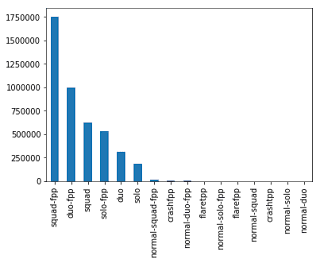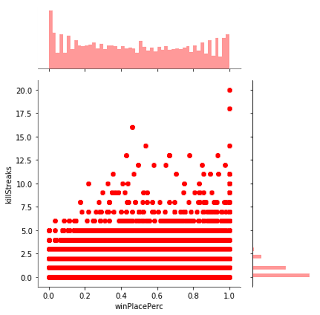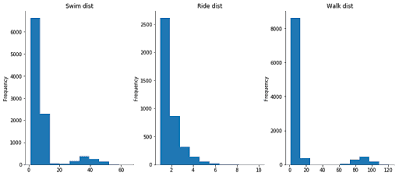### PUBG - Predicting win Percentage

All the game lovers will love this post. For those who don't know about PlayerUnknown's Battlegrounds popularly knows as PUBG is a multiplayer online game in which there are different missions or modes. Players can select there own maps. It tracks your kills, headshots and stuff like that. And If you do well and win the game you will get a Winner Winner Chicken Dinner. So in this post will be predicting the chances that a player wins (gets Winner Winner Chicken Dinner) in PUBG given different attributes. Let's get started.### Tools used and Dataset

The tools which we are going to use for this are:

• Python
• Scikit-learn
• Numpy and Pandas
• Pyplot and Seaborn
• Tensorflow and Keras (optional)

You can find the dataset here and the whole code for this here. I recommend you to use Kaggle kernel because the dataset is about 5 GB and has about 4.5 Million rows.

### Implementation

We are going to do this in 4 steps which are first data preparation, data exploration, data modeling (training and fitting of model) and lastly data validation. I am going to how to fit models using scikit-learn as well as TensorFlow and Keras. So let's start.

#### Data preparation

Let's start by preparing the data and removing the null rows. I think there is only one null value in our dataset but still, we have to remove that.

``````train = pd.read_csv("train_V2.csv")

#Remove null values
train.isna().sum()
train = train[train.winPlacePerc.isna() == False]
train.info()``````

By looking at the data we found that there are 25 columns which include stuff like matchId, assists, boosts, number of kills and many others. Our goal is to predict the Win Percentage. Now let's explore the data.

#### Data Exploration

First, let us look at the number of rows and various rows.

```train.shape
train.describe()```

So we found that there are 4446965 rows. Lets now explore the data furthermore using pyplot and seaborn.

```z = train.matchType.value_counts()
z.plot.bar()
plt.show()```
``

This shows the barplot of match type and we can see that there are about 1.75 million rows of squad-fpp (which I think means first player squad matches). Now let's look at the number of kills.

```z = train.kills.value_counts()
plt.figure(figsize=(12,5))
z.plot.bar()
plt.show()```
``

We can see that most of the players didn't kill anybody and the as the number increases the number of instances decreases with the highest being 72 (which is pretty amazing). Let us plot the relation between win percentage and killStreaks.

```sns.jointplot(x='winPlacePerc', y='killStreaks', data=train, ratio=3, color='r')
plt.show()```
``

We can see no specific pattern but there are still some values that show higher win place percentage.

```figure1, axarr1 = plt.subplots(1, 3, figsize=(14, 6))
train['swimDistance'].value_counts().sort_index()[1:20].plot.hist(ax=axarr1)
train['rideDistance'].value_counts().sort_index()[1:20].plot.hist(ax=axarr1)
train['walkDistance'].value_counts().sort_index()[1:20].plot.hist(ax=axarr1)
axarr1.set_title('Swim dist')
axarr1.set_title('Ride dist')
axarr1.set_title('Walk dist')
sns.despine()```
``

We can see that most of our data is skewed to the left which is obvious. But no problem we can work with this data as long as our prediction variable (winplaceperc) is not skewed. Now let's prepare the data. First let's remove the first three columns (because I don't think they will affect our model) and shuffling the data.

``````data = train.iloc[:,3:].values
data = shuffle(data)``````

Also lets convert matchType to integer from string.

```matchtype_le = preprocessing.LabelEncoder()
matchtype_le.fit(train.matchType.unique())
enc = matchtype_le.transform(train.matchType)
data.matchType = enc```

Lets split the target and predicting columns.

```X = data[:,:-1]
y = data[:,-1]
X_train, X_test, y_train, y_test = train_test_split(X, y, test_size=0.02) ```

This will make the target and predicting columns as well as split the dataset in testing and training dataset. I have kept the size equal to 0.02 which means 2% of the data because we have almost 4.5 million rows.

#### Data modeling

Now that we have prepared our data we can create our model. I will show you how to create models both with sklearn (scikit-learn) and TensorFlow (Neural networks). First lets make our model with scikit learn. I have used linear regression but you guys can try other model algorithms as well. But some algorithms like random forest, svm might take more time.

``````clf = LinearRegression()
clf.fit(X_train, y_train)``````

That's it we have created our first model. Now let's use TensorFlow and Keras to create a neural network. This is an optional part so you can skip this (but I highly recommend learning about these frameworks).

```model = tf.keras.Sequential([
tf.keras.layers.Dense(32, input_shape = , activation = 'relu'),
tf.keras.layers.Dense(64, activation = 'relu'),
tf.keras.layers.Dense(128, activation = 'relu'),
tf.keras.layers.Dense(256, activation = 'relu'),
tf.keras.layers.Dense(128, activation = 'relu'),
tf.keras.layers.Dense(64, activation = 'relu'),
tf.keras.layers.Dense(32, activation = 'relu'),
tf.keras.layers.Dense(1, activation = 'linear')
])

model.summary()
model.fit(X_train, y_train, epochs=10)```

Now that we have created our models we can move on to the validation part.

#### Model Validation

Let us now check how good our models are.

```train_predict = clf.predict(X_train)
train_msq = mean_squared_error(y_train, train_predict)
train_r2 = r2_score(y_train, train_predict)
print("Train:\nMean Squared Error :", train_msq, "\nR2 score :", train_r2)

test_predict = clf.predict(X_test)
test_msq = mean_squared_error(y_test, test_predict)
test_r2 = r2_score(y_test, test_predict)
print("\nTest:\nMean Squared Error :", test_msq, "\nR2 score :", test_r2)  ```
```Train:
Mean Squared Error : 0.015894585552960392
R2 score : 0.8317955086282278
Test:
Mean Squared Error : 0.015921824946516883
R2 score : 0.8316977099939594```

We can see we have quite low mean square error for both Train and Test set which is good. Also the R2 score is about 0.83 for both which is quite good considering the max is 1.

Now lets check the model we built on keras.

```train_predict = model.predict(X_train)
train_msq = mean_squared_error(y_train, train_predict)
train_r2 = r2_score(y_train, train_predict)
print("Train:\nMean Squared Error :", train_msq, "\nR2 score :", train_r2)

test_predict = model.predict(X_test)
test_msq = mean_squared_error(y_test, test_predict)
test_r2 = r2_score(y_test, test_predict)
print("\nTest:\nMean Squared Error :", test_msq, "\nR2 score :", test_r2)  ```
```Train:
Mean Squared Error : 0.0074
R2 score : 0.9170
Test:
Mean Squared Error : 0.007344114
R2 score : 0.917466```

We can see our Neural network performed far better than our linear regression model.

So in this post, we learned how to fit the linear model and neural network on PUBG dataset. You can find the whole code here.